（万字好文！）你可能需要的数据结构与算法最详细的入门教材了！

# （万字好文！）你可能需要的数据结构与算法最详细的入门教材了！

2021-08-01 9:14:4415

# 基础知识

## 栈

``````栈是一种遵从后进先出（LIFO）原则的数据结构。
``````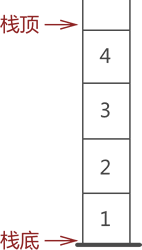如上图所示，栈顶和栈低，在上图中，我们可以清晰的看到，先入栈的数据由于被压在栈底，如果想要出栈，那么必须等前方的数字都出栈才能轮到他，于是就形成了我们的后进先出的数据结构（强调一下栈是一种数据结构）

``````	class Stack {
constructor() {
this.stack = []
// 栈的长度
this.size = 0
}
//  入栈
push(i) {
this.stack[this.size] = i
this.size++

}
// 出栈
pop() {
if (this.stack.length > 0) {
const last = this.stack[--this.size]
this.stack.length--
return last
}

}
// 查看栈顶元素
peek() {
return this.stack[this.size - 1];
}
// 栈是否为空
isEmpty() {
return stack.length === 0;
}
// 清空栈
clear() {
this.stack = []
}
// 查看栈元素
see(){
console.log(this.stack)
return this.stack
}
}
``````

## 队列

``````队列是一种遵从先进先出原则的数据结构。
``````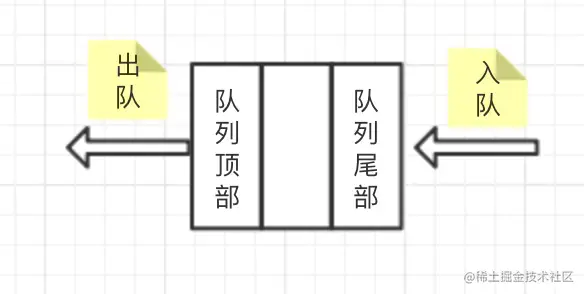如上图所示，我们发现最先进入的数据，只能先出去，他具有先进先出的特性。然而，在js的语法中同样的没有队列的数据结构，但是我们依然可以用数据来模拟队列的数据结构，由于队列是先进先出，那么，也就是如上图所示，我们首先需要模拟原生的入队push方法，再模拟原生的shift方法，ok 废话少说开始吧！

``````	class Queue {
constructor() {
// 创建一个队列
this.queue = []
// 队列长度
this.size = 0
}
//  入队列
push(i) {
this.queue[this.size] = i
this.size++

}
// 出队列
shift() {
if (this.queue.length === 0) {
return
}
const first = this.queue
//将后面的赋值给前面的
for (let i = 0; i < this.queue.length - 1; i++) {
this.queue[i] = this.queue[i + 1]
}
this.queue.length--
return first
}
//获取队首
getFront() {
return this.queue;
}
//获取队尾
getRear() {
return this.queue[this.size - 1]
}
// 清空队列
clear() {
this.queue = []
}
// 查看队列元素
see() {
console.log(this.queue)
return this.queue
}
}
``````

## 链表

``````用链式存储的线性表统称为链表
``````

### 单向链表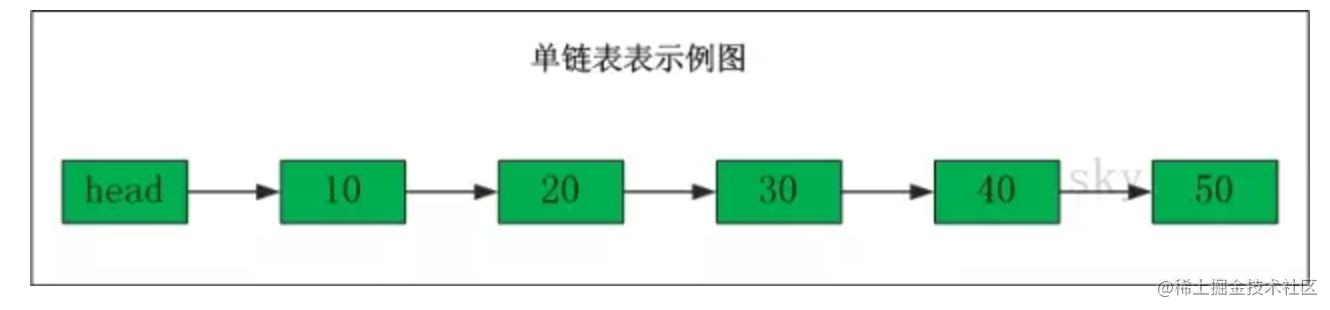如上图所示，就是一个简单的单项链表，单向链表(单链表)是链表的一种，它由节点组成，每个节点都包含下一个节点的指针，下图就是一个单链表，表头为空，表头的后继节点是"结点10"(数据为10的结点)，"节点10"的后继结点是"节点20"(数据为10的结点)

### 双向链表!

``````var a = { name: '大佬1' }
var b = { name: '大佬1' }
var c = { name: '大佬1' }
var d = { name: '大佬1' }
var e = { name: '大佬1' }
// 建立链表
a.next=b
b.next=c
c.next=d
d.next=e
``````

``````// 遍历链表
let p = a
while (a) {
console.log(a)
p = a.next()
}
// 插入链表
const f={name:'大佬1'}
//模拟在a元素后插入
a.next=f
f.next=b

// 删除链表
// 在删除f
a.next=b
``````

## 集合

``````集合是由一组无序但彼此之间又有一定相关性的成员构成的, 每个成员在集合中只能出现一次.他是一个无序且唯一的数据结构
``````

### Set

set是es6新出的一种数据结构，Set对象是值的集合，你可以按照插入的顺序迭代它的元素。 Set中的元素只会出现一次，即 Set 中的元素是唯一的。接下来让我们来看看set的基本操作

``````// 创建一个 集合
var s = new Set([1, 2, 3, '3', 4]);
//添加一个key
//重复元素在Set中自动被过滤
console.log(s);//Set {1, 2, 3, 4,5}
//删除一个key
s.delete(2);
// 判断是否有这个元素
s.has(3)
console.log(s);//Set{1, 3, "3", 4, 5}//注意数字3和字符串'3'是不同的元素。
``````

``````// 并集
const  arr1=new Set([1,2,4])
const arr2=new Set([2,3,4,5])
new Set([...arr1, ...arr2])
//交集
const arr1=new Set([1,3,4,6,7,9])
const arr2=new Set([2,3,4,5,6,7])
const arr=new Set([...arr1].filter((item)=>{arr2.has(item)}))
``````

## 字典

``````字典 是一种以键-值对形式存储唯一数据的数据结构
``````

### Map

``````// 创建一个字典
const map=new Map([
['a',1],
['b',2]
]);
console.log(map);
``````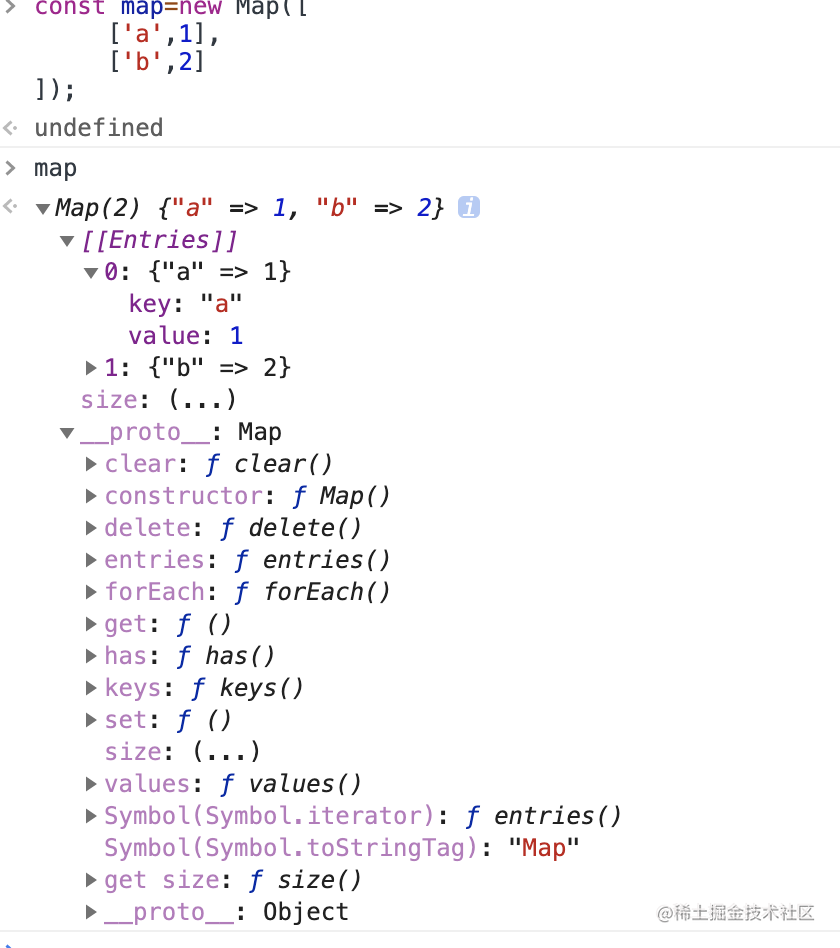上图所示，就是我们的字典的数据结构，你发现他的原型不同，并且存储结构也不同，那么有的同学又问了，都有了对象了，而且能实现Map的功能，为啥还要开发一个Map，这里我的理解是，为了让对象的功能和作用更纯粹，从而使得这门语言规范法，标准化，以及打开变函数式编程的大门，接下来，我们来简单的使用字典的增删改查

`````` // 字典的增删改查
// 获取长度
console.log(map.size);
// 增加key 可以是个非原始类型
console.log(map.set([1,2],''));
map.set('name','李丽').set('age',18);
// 查询
console.log(map.get('name'));
// 删除
console.log(map.delete('name'));
// 是否含有
console.log(map.has('name'));

``````

`````` //  求最大的三个数 并且求出下标
// =======解题思路=======
//1、采用的是暴力求解法，那么我们可以利用字典的特点存储最大的三个值
//2、还有种比较好理解的办法利用排序，在之前文章里写过
function maxThree(arr) {
var i = 0;
var j = 0;
var tmp = arr
var v = 0
var b = new Map()
// 遍历三次分别找到最大的三个值
while (i < 3) {
while (j < arr.length) {
if (tmp < arr[j] && !b.has(j)) {
tmp = arr[j]
v = j
}
j++
}

b.set(v, tmp)
i++
j = 0
tmp = 0
}
// console.log(b)
}
maxThree([1, 3, 4, 5, 6, 7, 9, 1, 1, 9, 2, 8, 3, 4, 5,])
``````

## 图

``````图是一种网络结构的抽象模型，它是一组由边连接的顶点组成
``````

### 邻接矩阵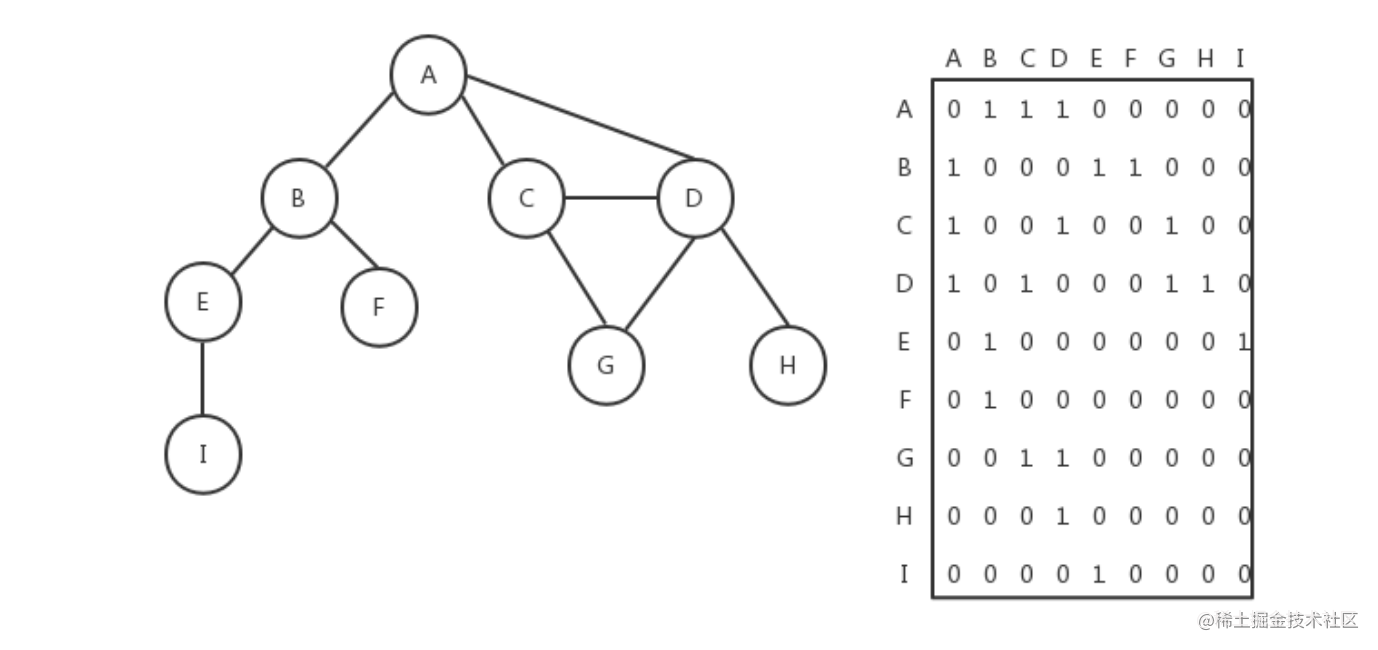如上图所示我们就将一个图抽象成了邻接矩阵表示，其实本质就是用一个二维数组去表示连接点之间的关系，好，废话少说，我们接下来用邻接矩阵代码去表示一个简单的图

``````var matrix=[
[0,1,0,0,0],
[0,0,1,1,0],
[0,0,0,0,1],
[1,0,0,0,0],
[0,0,0,1,0]
]
``````

### 邻接表

``````  class Graph {
constructor() {
this.vertices = []; // 用来存放图中的顶点
this.adjList = new Map(); // 用字典的数据结构来存放图中的边
}

// 向图中添加一个新顶点
if (!this.vertices.includes(v)) {
this.vertices.push(v);
}
}

// 向图中添加a和b两个顶点之间的边
// 如果图中没有顶点a，先添加顶点a
}
// 如果图中没有顶点b，先添加顶点b
}

}
}
let graph = new Graph();
let myVertices = ['A', 'B', 'C', 'D', 'E'];
myVertices.forEach((v) => {
});

console.log(graph);
``````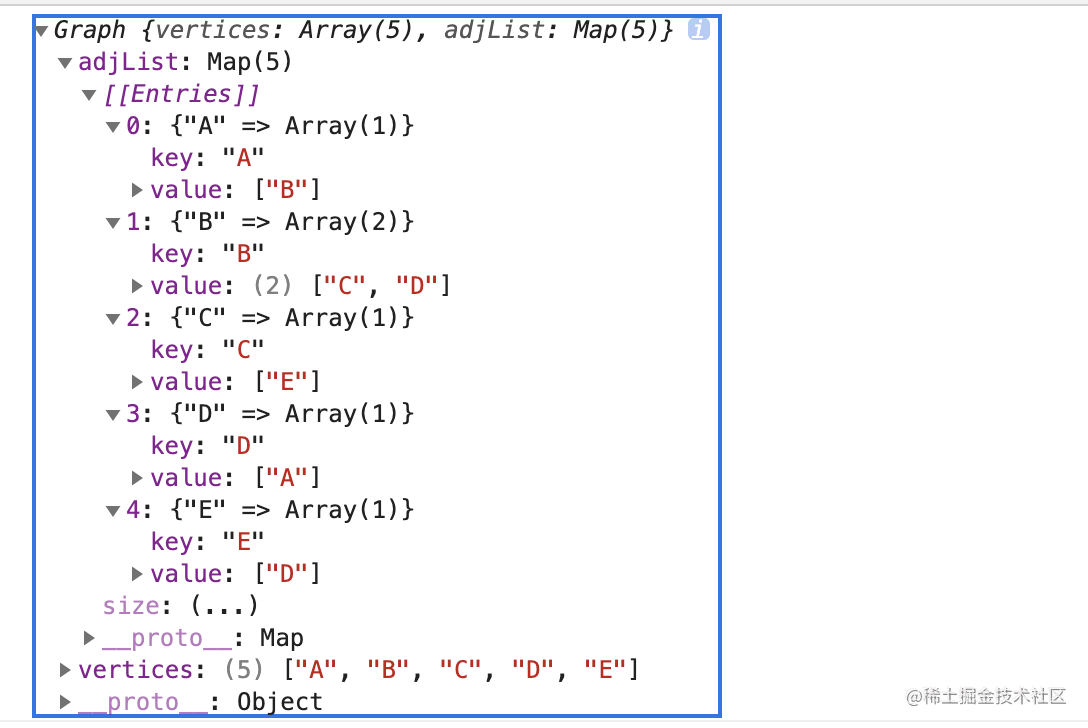如图，邻接表也完整映射出图的关系

## 树

``````在计算机科学中，树是一种十分重要的数据结构。树被描述为一种分层数据抽象模型，常用来描述数据间的层级关系和组织结构。树也是一种非顺序的数据结构。
``````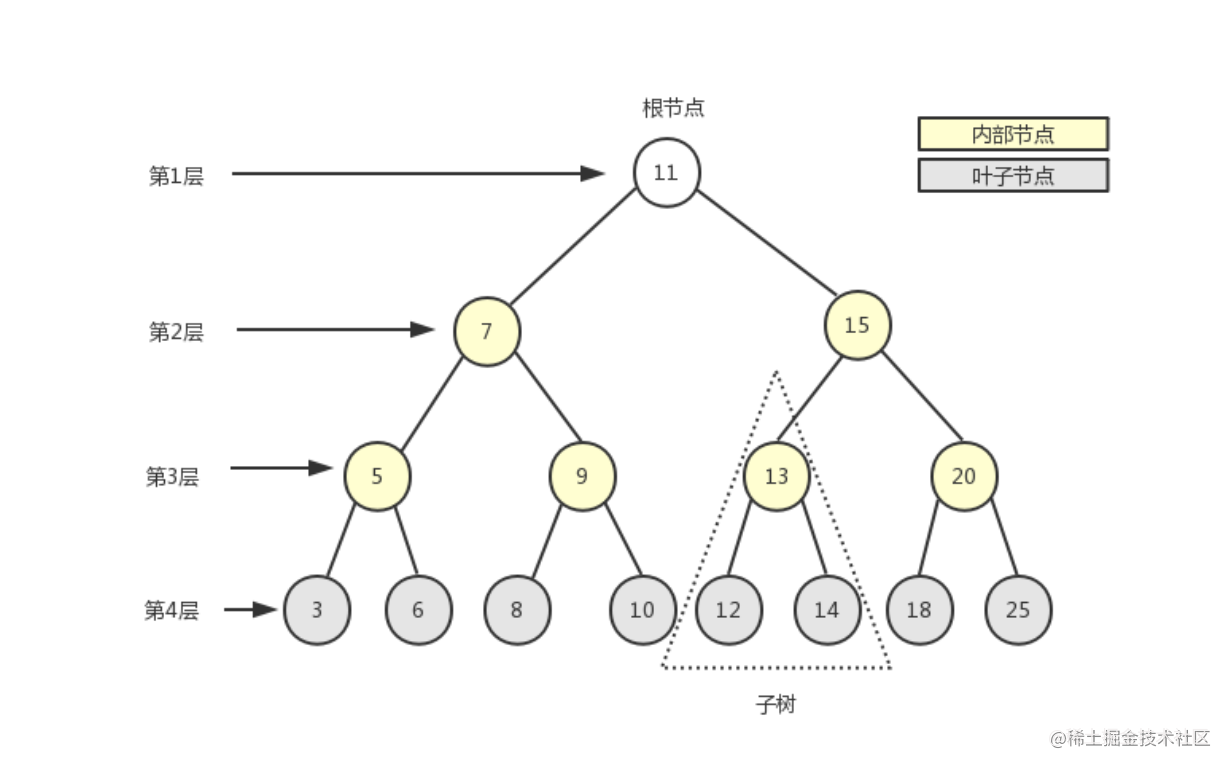如上图所示，这就是一个树形象展示，而在我们前端中，其实是和树打交道最多的了，比如dom树、级联选择、属性目录控件这是我们最常听见的名词了吧，他其实就是一个树的数据结构。在我们前端js中同样的也没有树的数据结构。但是我们可以用对象和数组去表示一个树。

`````` var tree={
value:1,
children:[
{
value:2,
children:[
{
value:3
}
]
}
]
}
``````

### 深度优先遍历（DFS）

``````div=>ul=>li=>a=>img=>li=>span=>li=>p=>button
``````

`````` //将dom 抽象成树
var dom = {
tag: 'div',
children: [
{
tag: 'ul',
children: [
{
tag: 'li',
children: [
{
tag: 'a',
children: [
{
tag: 'img'
}
]
}

]
},
{
tag: 'li',
children: [
{
tag: 'span'
}
]
},
{
tag: 'li'
}
]
},
{
tag: 'p'
},
{
tag: 'button'
}
]
}
var nodeList = []
//深度优先遍历算法
function DFS(node, nodeList) {
if (node) {
nodeList.push(node.tag);
var children = node.children;
if (children) {
for (var i = 0; i < children.length; i++) {
//每次递归的时候将 需要遍历的节点 和 节点所存储的数组传下去
DFS(children[i], nodeList);
}
}
}
return nodeList;
}
DFS(dom, nodeList)
console.log(nodeList)
``````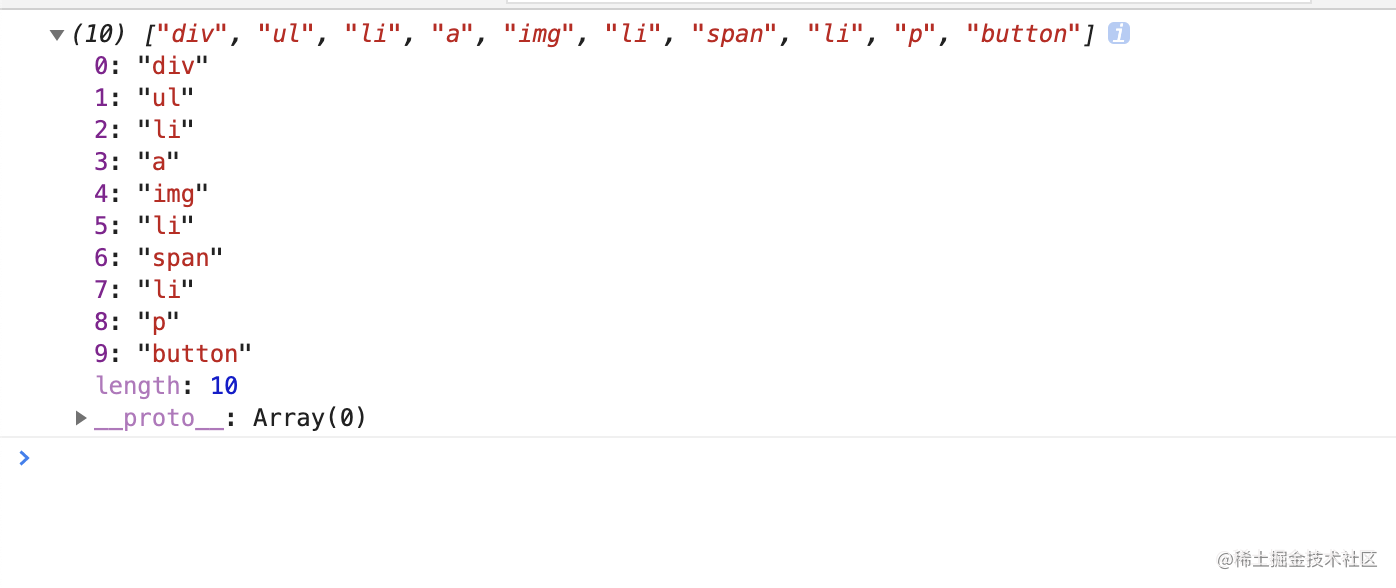结果也相当的一致

### 广度优先遍历（BFS）

``````div=>ul=>p=>button=>li=>li=>li=>a=>span=>img
``````

``````        function BFS(node, nodeList) {
//由于是广度优先，for循环不是很优雅，我们可以使用队列来解决
if (node) {
var q = [node]
while (q.length > 0) {
var item = q.shift()
nodeList.push(item.tag)
if (item.children) {
item.children.forEach(e => {
q.push(e)
})
}

}
}
}
BFS(dom, nodeList)
console.log(nodeList)``

``````

### 二叉树

``````二叉树(Binary Tree)是一种树形结构，它的特点是每个节点最多只有两个分支节点，一棵二叉树通常由根节点，分支节点，叶子节点组成。而每个分支节点也常常被称作为一棵子树。
``````

``````const bt = {
val: 1,
left: {
val: 2,
left: {
val: 4,
left: null,
right: null,
},
right: {
val: 5,
left: null,
right: null,
},
},
right: {
val: 3,
left: {
val: 6,
left: null,
right: null,
},
right: {
val: 7,
left: null,
right: null,
},
},
}
``````

#### 创建二叉树

``````  // arr=[6,5,6,8,9,1,4,3,6] 将数组根据下标为0的大小转换成二叉树
arr = [6, 5, 6, 8, 9, 1, 4, 3, 6]
class BinaryTreeNode {
constructor(key) {
// 左节点
this.left = null;
// 右节点
this.right = null;
// 键
this.key = key;
}
}
class BinaryTree {
constructor() {
this.root = null;
}
insert(key) {
const node = new BinaryTreeNode(key)
if (this.root === null) {
this.root = node
} else {
this.insertNode(this.root, node)
}
}
// 抽离递归比较部分逻辑
insertNode(node, newNode) {
if (node.key < newNode.key) {
if (node.left) {
this.insertNode(node.left, newNode)
} else {
node.left = newNode
}

} else {
if (node.right) {
this.insertNode(node.right, newNode)
} else {
node.right = newNode
}
}
}
}
const tree = new BinaryTree();
arr.forEach(key => {
tree.insert(key)
});
console.log(tree)
``````

#### 中序遍历

``````中序遍历(inorder)：先遍历左节点，再遍历自己，最后遍历右节点，输出的刚好是有序的列表
中序遍历 有递归版本和 非递归的版本，此处使用递归版本
// 是对象中的一个方法
inorderTransverse(root, arr) {
if (!root) return;
this.inorderTransverse(root.left, arr);
arr.push(root.key);
this.inorderTransverse(root.right, arr);
}
// 使用
const arrTransverse = []
tree.inorderTransverse(tree.root, arrTransverse)
console.log(arrTransverse)
``````

#### 先序遍历

``````先序遍历(preorder)：先自己，再遍历左节点，最后遍历右节点
preorderTransverse(root, arr) {
if (!root) return;
arr.push(root.key);
this.preorderTransverse(root.left, arr);
this.preorderTransverse(root.right, arr);
}
``````

#### 后序遍历

``````后序遍历(postorder)：先左节点，再右节点，最后自己
// 由于后序遍历的非递归版本比较巧妙，我们使用非递归版本
postorderTransverse(root, arr) {
// 创建一个栈
var stack = [];
// 将根节点压入栈顶
stack.push(root);
while (stack.length > 0) {
let node = stack.pop();
// 利用unshift按照顺序压入数组
arr.unshift(node.key);
if (node.left) {
stack.push(node.left);
}
if (node.right) {
stack.push(node.right);

}
}
}
// 调用
const arrTransverse = []
tree.postorderTransverse(tree.root, arrTransverse)
``````

### 真题演练

`````` //      1
//    2   3
//  4  5 6 7
// 将如上二叉树翻转过来
//===== 解题思路======
//1、如果想翻转二叉树，使用分治思想是一种比较好理解的方式
//2、主要就是递归每一层，递归到当前层只需要交换当前层的二叉树即可
function invertTree(bt) {
if (!bt) {
return bt
}
//利用解构赋值交换两个数
[bt.left, bt.right] = [invertTree(bt.right), invertTree(bt.left)];
return bt
}
console.log(invertTree(bt))
``````

## 堆

``````堆是什么? 在前端中甚至都很少提过这个概念，其实，堆是一种特殊的完全二叉树
``````

``````若设二叉树的深度为k，除第 k 层外，其它各层 (1～k-1) 的结点数都达到最大个数，第k 层所有的结点都连续集中在最左边，这就是完全二叉树。
``````

• 所有的节点都大于等于或者小于等于它的子节点
• 如果每个节点都大于等于它的子节点是最大堆
• 如果每个节点都小于等于它的子节点是最小堆

``````   class MinHeep {
constructor() {
this.heap = []
}
// 插入方法
insert(val) {
this.heap.push(val)
this.shiftUP(this.heap.length - 1)

}
// 交换方法
swap(i, v) {
var temp = this.heap[i]
this.heap[i] = this.heap[v]
this.heap[v] = temp
}
// 上移方法
shiftUP(index) {
if (index === 0) { return }
var preIindex = this.getParentIndex(index)
if (this.heap[preIindex] > this.heap[index]) {
this.swap(preIindex, index)
}
}
getParentIndex(i) {
// 求商的方法
return (i - 1) >> 1
}

}
var h=new MinHeep()
h.insert(3)
h.insert(1)
h.insert(2)
h.insert(7)
h.insert(4)
console.log(h.heap)
``````

## 时间复杂度空间复杂度

``````时间维度：是指执行当前算法所消耗的时间，我们通常用「时间复杂度」来描述。

``````

### 表示方法

``````var a=1
a++
``````

• 常数阶O(1)
• 对数阶O(logN)
• 线性阶O(n)
• 线性对数阶O(nlogN)
• 平方阶O(n²)
• 立方阶O(n³)
• K次方阶O(n^k)
• 指数阶(2^n)

``````for(i=1; i<=n; ++i)
{
j = i;
j++;
}
``````

``````int i = 1;
while(i<n)
{
i = i * 2;
}
``````

``````//O(1)
var a=1
a++
// 声明数组，有n个元素O(n)
var a=new Array(n)

``````

# 高级思想

## 排序算法

### 冒泡排序

1、比较相邻的元素。如果第一个比第二个大，就交换他们两个。 2、对每一对相邻元素作同样的工作，从开始第一对到结尾的最后一对。这步做完后，最后的元素会是最大的数。 3、针对所有的元素重复以上的步骤，除了最后一个。 4、持续每次对越来越少的元素重复上面的步骤，直到没有任何一对数字需要比较。

``````function bubble(arr){
let tem = null;
//外层i控制比较的轮数
for(let i=0;i<arr.length;i++){
// 里层循环控制每一轮比较的次数
for(let j=0;j<arr.length-1-i;j++){
if(arr[j]>arr[j+1]){
//当前项大于后一项,交换位置
tem = arr[j];
arr[j] = arr[j+1];
arr[j+1] = tem;
}
}
}
return arr
}
``````

### 选择排序

1、首先在未排序序列中找到最小（大）元素，存放到排序序列的起始位置 2、再从剩余未排序元素中继续寻找最小（大）元素，然后放到已排序序列的末尾。 3、重复第二步，直到所有元素均排序完毕。

``````//先选择出最小的值组个比较调换位置
function selectSort(arr){
let length = arr.length;
for(let i=0;i<length-1;i++){
let min = i;
for(let j=min+1;j<length;j++){
if(arr[min]>arr[j]){
min = j
}
}
if(min!=i){
let temp = arr[i];
arr[i] = arr[min];
arr[min] = temp;
}
}
return arr
}
``````

### 插入排序

1、从第二个数字往前比。 2、比他大的往后排，以此类推直接排到末尾

``````function insertSort(arr) {
let length = arr.length;
for(let i = 1; i < length; i++) {
let temp = arr[i];
let j = i;
for(; j > 0; j--) {
if(temp >= arr[j-1]) {
break;      // 当前考察的数大于前一个数，证明有序，退出循环
}
arr[j] = arr[j-1]; // 将前一个数复制到后一个数上
}
arr[j] = temp;  // 找到考察的数应处于的位置
}
return arr;
}

// example
let arr = [2,5,10,7,10,32,90,9,11,1,0,10]
console.log(insertSort(arr));
``````

### 归并排序

1、把数组劈成两半，在递归的对子数组进行劈开的操作，知道分成一个个单独的数 2、把两个数组合并成有序数组，在对有序数组进行合并直到全部子数组合并为一个完整数组

``````    function mergeSort(arr) {
// 劈开数组
if (arr.length === 1) { return arr }
const mid = Math.floor(arr.length / 2)
const left = arr.slice(0, mid)
const right = arr.slice(mid, arr.length)
// 递归
const orderLeft = mergeSort(left)
const orderRight = mergeSort(right)

const res = []
while (orderLeft.length || orderRight.length) {
// 利用队列排序数字并压入数组
if (orderLeft.length && orderRight.length) {
res.push(orderLeft < orderRight ?
orderLeft.shift() : orderRight.shift())
} else if (orderLeft.length) {
res.push(orderLeft.shift())
} else if (orderRight.length) {
res.push(orderRight.shift())
}
}
return res
}
var arr = [1, 4, 6, 7, 9, 5]
console.log(mergeSort(arr))
``````

### 快速排序

1、首先需要分区，从数组送任意选择一个基准，然后将前后的值跟跟基准去比较，如果比基准小，那么放入左边数组，否则放入右边数组 2、递归的对子数组进行分区知道最后和合并排序号的子数组

`````` var arr = [2, 4, 3, 5,  1]
function quickSort(arr) {
if (arr.length === 1 || arr.length === 0) { return arr }
const left = [], right = []
// 找到基准,暂时取下标0
const mid = arr
// 注意由于0被取了，从1开始
for (let i = 1; i < arr.length; i++) {
if (arr[i] < mid) {
left.push(arr[i])
} else {
right.push(arr[i])
}
}
// 递归
console.log(left, right)
return [...quickSort(left), mid, ...quickSort(right)]
}
console.log(quickSort(arr))
``````

## 搜索算法

### 顺序搜索

``````// 挂载原型上不用传两个值
Array.prototype.sequentialSearch = function(item) {
for(let i = 0; i < this.length; i+=1) {
if(this[i] === item) {
return i;
}
}
return -1;
};

const res = [1,2,3,4,5].sequentialSearch(3)
console.log(res);
``````

### 二分搜索

``````  Array.prototype.binarySearch=function( key) {
var low = 0;
var high = this.length - 1;

while (low <= high) {
var mid = parseInt((low + high) / 2);

if (key === this[mid]) {
return mid;
}
else if (key < this[mid]) {
high = mid - 1;
}
else if (key > this[mid]) {
low = mid + 1;
}
else {
return -1;
}
}
}

var arr = [1,2,3,4,5,6,7,8];
console.log(arr.binarySearch(3));
``````

## 分治思想

``````他其实就是将一个待解决的问题分解成多个小问题递归解决，再将结果合并以解决原来的问题
``````

## 动态规划

``````动态规划和分而治之类似，也是算法设计中的一种方法，同样的他也是将一个问题分解成相互重叠的子问题，通过去求解子问题，来达到求解原来的问题的目的
``````

### 斐波那契数列

`````` 斐波那契数列（Fibonacci sequence），又称黄金分割数列、因数学家莱昂纳多·斐波那契（Leonardoda Fibonacci）以兔子繁殖为例子而引入，故又称为“兔子数列”，指的是这样一个数列：0、1、1、2、3、5、8、13、21、34
``````

### 真题演练

`````` //假设你正在爬楼梯。需要 n 阶你才能到达楼顶。
//每次你可以爬 1 或 2 个台阶。你有多少种不同的方法可以爬到楼顶呢？ 注意：给定 n 是一个正整数。
// 示例
// 输入： 2
// 输出： 2
// 解释： 有两种方法可以爬到楼顶。
// 1.  1 阶 + 1 阶
// 2.  2 阶
// =======分析=========
// 首先我们假设只有3阶，那么我先定义好，我最后一步可以是两阶，也可能是一阶，
// 如果最后一步是两阶,我们就能知道之前就只有一种方法才能达到最后一步是两阶梯
// 如果最后一步是一阶我们就是知道之前有两种方法可以到达最后一阶梯
// 由于我们的最后一步的阶梯数定死了，所以，他的阶梯数量就是之前的方法之和
// 如此我们就可以推导出f(n)=f(n-1)+f(n-2) 公式
var climbStairs = function (n) {
// 由于我们的公式是f(n)=f(n-1)+f(n-2),所有当n小于2的时候我们直接返回，兼容边界条件
if (n < 2) { return 1 }
// 我们先定义初始的能求值的数组,下标0为1是由于我们只有一阶的时候也只有一种方法
const dp = [1, 1]
//套用公式
for (let i = 2; i < n; i++) {
dp[i] = dp[i - 1] + dp[i - 2]
}
// 返回当前阶梯的方法
return dp[n]
};
``````

## 贪心算法

``````贪心算法也是算法设计中的一种方法，他是通过每个阶段的局部最优选择，从而达到全局最优
``````

ok，接下来举个例子，我们有1，2，5三种面值硬币，现在要求是我们使用最少的面值硬币来凑成11块钱，那如果使用贪心算法，我们为了使用的硬币最少，上来是不是需要选个5，接着在选个5，最后选个1，如此一来我们只需要三个硬币就能凑齐11块钱，当然在这种情况下，他就是最优解，那么如果我们给硬币换一下，我们需要1，3，4三种面值硬币，我们需要凑够6块钱，如果按照贪心算法，那么 组合就是4+1+1， 然而实际的最优解确实3+3只需要两个就可以了。

### 真题演练

``````//定一个数组，它的第 i 个元素是一支给定股票第 i 天的价格。
// 设计一个算法来计算你所能获取的最大利润。你可以尽可能地完成更多的交易（多次买卖一支股票）。
// 注意：你不能同时参与多笔交易（你必须在再次购买前出售掉之前的股票）。
// 举例输入: [7,1,5,3,6,4] 输出: 7
//解释: 在第 2 天（股票价格 = 1）的时候买入，在第 3 天（股票价格 = 5）的时候卖出, 这笔交易所能获得利润 = 5-1 = 4 。
//随后，在第 4 天（股票价格 = 3）的时候买入，在第 5 天（股票价格 = 6）的时候卖出, 这笔交易所能获得利润 = 6-3 = 3 。S
//======= 解题思路 =========
// 1、由于我们在开始之初就能拿到股票的走势数组，那么也就是我们已知股票的结果
// 2、既然已知股票结果，那么利用贪心算法的原则，我们只考虑局部最优解
// 3、只需要遍历整个数组发现是上涨的交易日，我们就进行买卖操作，下降的则不动，这样永远不会亏损
// 4、使用贪心算法的思路就是不管怎样，我不能赔钱，这样做的好处就是容易理解，如果使用动态规划，还要总结公式
var maxProfit = function (prices) {
var profit = 0
for (var i = 1; i < prices.length; i++) {
tmp = prices[i] - prices[i - 1]
//只有上涨我才买卖
if (tmp > 0) {
profit += profit
}
}
return profit
};
``````

## 回溯算法

``````回朔算法也是算法设计当中的一种思想，是一种渐进式寻找并构建问题解决方式的一种策略
``````

1、用递归模拟所有的情况

2、遇到包含重复元素的情况，就返回上一步（官话叫做回溯）

3、搜集并返回所有的没有重复的顺序

``````//给定一个 没有重复 数字的序列，返回其所有可能的全排列。
//输入: [1,2,3]
//输出:
//[
//[1,2,3],
//[1,3,2],
//[2,1,3],
//[2,3,1],
//[3,1,2],
//[3,2,1]
//]
//=========题目解析===========
//1、采用回溯算法求解
//2、将不重复数字一次放入数组的每个位置，如果满足条件，取出来，否则回溯寻找下一组
//3、使用递归实现回溯思路
const permute = (nums) => {
const res = [];
var dfs = function (path) {
if (path.length === 3) { res.push(path); return }
nums.forEach(element => {
if (path.includes(element)) { return }
// 多层递归实现回溯
dfs(path.concat(element))
});
}
dfs([])
console.log(res)
};
permute([1, 2, 3])
``````

## 一些套路

### 滑动窗口、双指针

``````// 无重复最长子串
//输入: s = "abcabcbb"
//输出: 3
//解释: 因为无重复字符的最长子串是 "abc"，所以其长度为 3。
//=======解题思路========
// 1、 这种题目是需要有解提套路的，比如，使用双指针，比如使用滑动窗口
var lengthOfLongestSubstring = function (s) {
var l = 0, r = 0, max = 0;// 建立两个指针先指向下标0
const mapObj = new Map()
while (r < s.length) {
// 两层判断防住重复元素不在滑动窗口内的情况
if (mapObj.has(s[r]) && mapObj.get(s[r]) >= l) {
l = mapObj.get(s[r]) + 1
}
max = Math.max(r - l + 1, max)
mapObj.set(s[r], r)
r++
}
console.log(max)
};
lengthOfLongestSubstring('djcqwertyuhjjkkiuy')
``````

### 善于利用Map

``````   //给定一个整数数组 nums 和一个目标值 target，请你在该数组中找出和为目标值的那 两个 整数，并返回他们的数组下标。
//你可以假设每种输入只会对应一个答案。但是，数组中同一个元素不能使用两遍。

//给定 nums = [2, 7, 11, 15], target = 9
//因为 nums + nums = 2 + 7 = 9
//所以返回 [0, 1]
//=======解题思路=========
//1、 本题有暴力解法和非暴力解法
//2、 非暴力解法如果不是有人提拔，一般人很难想到
//3、 我们需要想象我们找到目结果就是凑对，相当于婚介所找对象
//4、 思路就是来的元素全部存档，当有别的元素进来时，在已存档的元素中去凑出目标结果
var twoSum = function (nums, target) {
var obj = new Map()
nums.forEach((item, i) => {
// 如果找到返回
if (obj.has(nums[i])) {

console.log([obj.get(nums[i]), i])
} else {
// 记录对象,并存储下标
obj.set(target - nums[i], i)
}
})
};
twoSum([2, 7, 11, 15], 18)
``````

### 快慢指针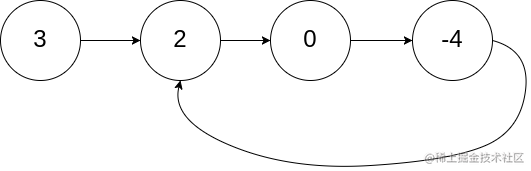``````  // 判断是否是环形链表
//输入：head = [3,2,0,-4], pos = 1
//输出：true
//解释：链表中有一个环，其尾部连接到第二个节点。
//========解题思路==========
//1、在我最初的时候也是暴力求解法也是正常人的思维所能想到的
//2、历所有节点，每次遍历到一个节点时，判断该节点此前是否被访问过。
//3、然而我看了大佬们的清奇的解题思路震惊了，估计想几天也想不到
//4、他们使用的就是快慢指针其实也是双指针
//5、思路就是建立两个指针，一个每次走两个next，一个每次走一个next ，如果最后两个相遇，说明一定是环形链表
/**
* @return {boolean}
*/
val: 3
}
val: 2
}
val: 0
}
val: -4
}
var hasCycle = function (head) {
// 如果是环形指针就用户按递归下去直到retrun 跳出函数
// 如果不是环形指针就会有尽头，这是最巧妙的地方
while (fast && fast.next) {
slow = slow.next
fast = fast.next.next
// 表示相遇了，返回环形指针
if (slow == fast) {
console.log(true)
return true
}

}
console.log(false)
return false
};
``````

# 最后苏公网安备 32030302000848号   苏ICP备20033101号-1